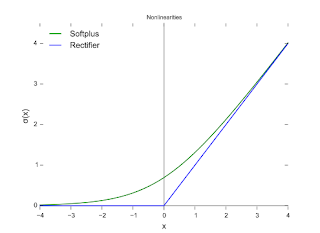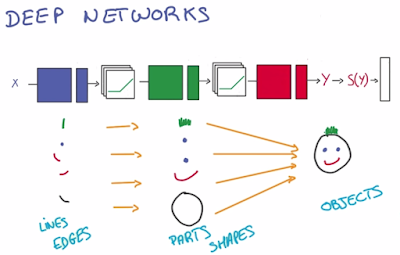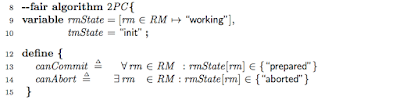## Wednesday, January 11, 2017

### Learning Machine Learning: Deep Neural Networks

This post is part of the ML/DL learning series. Earlier in the series, we covered these:
+ Learning Machine Learning: A beginner's journey
+ Linear Regression
+ Logistic Regression
+ Multinomial Logistic Regression

In this part, we are going to add hidden layers to our neural network, learn how backpropagation works for gradient descent in a deep NN, and finally talk about regularization techniques for avoiding overfitting.

For this post also, I follow the course notes from the Udacity Deep Learning Class by Vincent Vanhoucke at Google. Go, take the course. It is a great course to learn about deep learning and TensorFlow.

## Linear models are limited

We constructed a single layer NN for multinomial regression in our last post. How many parameters did that NN have? For an input vector X of size N, and K output classes, you have (N+1)*K parameters to use. N*K is the size of W, and K is the size of b.

You will need to use many more parameters in practice. Deep learning craves for big model as well as big data. Adding more layers to our NN will give us more model parameters, and enable our deep NN to capture more complex functions to fit the data better.

However, adding another layer that does linear matrix multiplication does not help much. With using just linear layers our NN is unable to efficiently capture nonlinear functions to fit the data. The solution is to introduce non-linearities at the layers via rectified linear units (ReLUs). Using ReLU layers r we get a layering of the form, Y= W1 r W2 r W3 X= WX. This lets us to use big weight matrix multiplications putting our GPUs to good use, enjoying numerically stable and easily derivativable linear functions, as well as seeping in some nonlinearities.

If you like to get a more intuitive neural-perspective understanding of NN, you may find this free book helpful.

## Rectified Linear Units (ReLUs)

ReLUs are probably the simplest non-linear functions. They're linear if x is greater than 0, and they're 0 everywhere else. RELUs have nice derivatives, as well. When x is less than zero, the value is 0. So, the derivative is 0 as well. When x is greater than 0, the value is equal to x. So, the derivative is equal to 1.We had constructed a NN with just the output layer for classification in the previous post. Now let's insert a layer of ReLUs to make it non-linear. This layer in the middle is called a hidden layer. We now have two matrices. One going from the inputs to the ReLUs, and another one connecting the ReLUs to the classifier.## Backpropagation

If you have two functions where one is applied to the output of the other, then the chain rule tells you that you can compute the derivatives of that function simply by taking the product of the derivatives of the components. $[g(f(x))]' = g'(f(x))*f'(x)$. There is a way to write this chain rule that is very computationally efficient.When you apply your data to some input x, you have data flowing through the stack up to your predictions y. To compute the derivatives, you create another graph that flows backwards through the network, get's combined using the chain rule that we saw before and produces gradients. That graph can be derived completely automatically from the individual operations in your network. Deep learning frameworks will do this backpropagation automatically for you.This backpropagation idea is explained beautifully (I mean it) here.

## Training a Deep NN

So to run stochastic gradient descent (SGD), for every single little batch of data in your training set, the deep NN

• runs the forward prop, and then the back prop and obtains the gradients for each of the weights in the model,
• then applies those gradients to the original weights and updates them,
• and repeats that over and over again until convergence.

You can add more hidden ReLU layers and make your model deeper and more powerful. The above backpropagation and SGD optimization applies the same to deeper NNs. Deep NNs are good at capturing hierarchical structure.## Regularization

In practice, it is better to overestimate the number of layers (and thus model parameters) needed for a problem, and then apply techniques to prevent overfitting. The first way to prevent overfitting is by looking at the performance under validation set, and stopping to train as soon as we stop improving.

Another way is to prevent overfitting is to apply regularization. For example in L2 Regularization, the idea is to add another term to the loss, which penalizes large weights.Another important technique for regularization that emerged recently is the dropout technique. It works very well and is widely used. At any given training round, the dropout technique randomly drops half of the activations that's flowing through the network and just destroy it. (The values that go from one layer to the next are called activations.) This forces the deep NN to learn a redundant representation for everything to make sure that at least some of the information remains, and prevents overfitting.